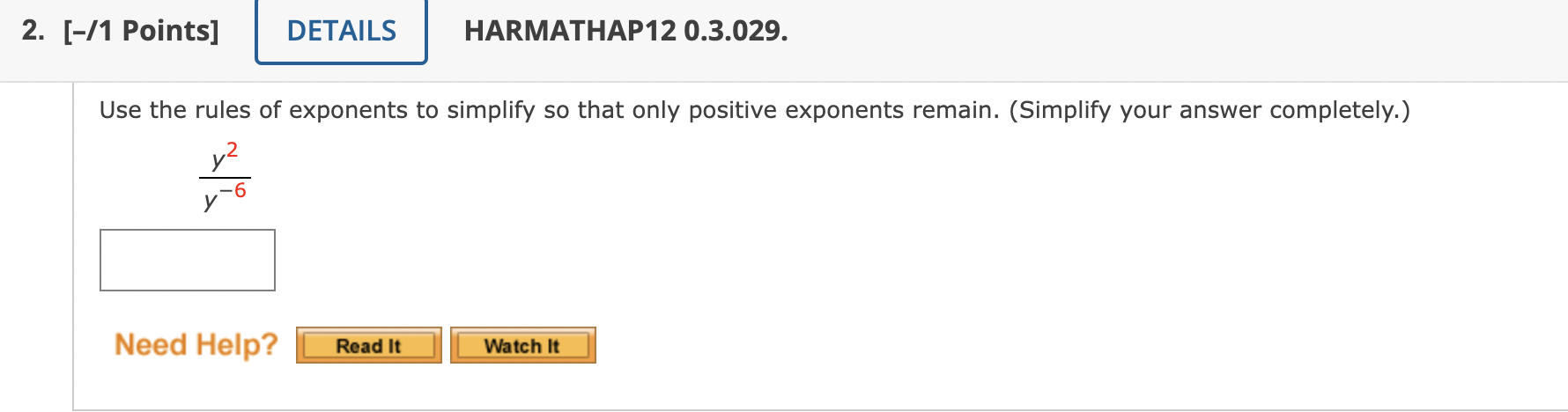Home / Expert Answers / Algebra / use-the-rules-of-exponents-to-simplify-so-that-only-positive-exponents-remain-simplify-your-answ-pa322

# (Solved): Use the rules of exponents to simplify so that only positive exponents remain. (Simplify your answ ...Use the rules of exponents to simplify so that only positive exponents remain. (Simplify your answer completely.) $\frac{y^{2}}{y^{-6}}$

We have an Answer from Expert